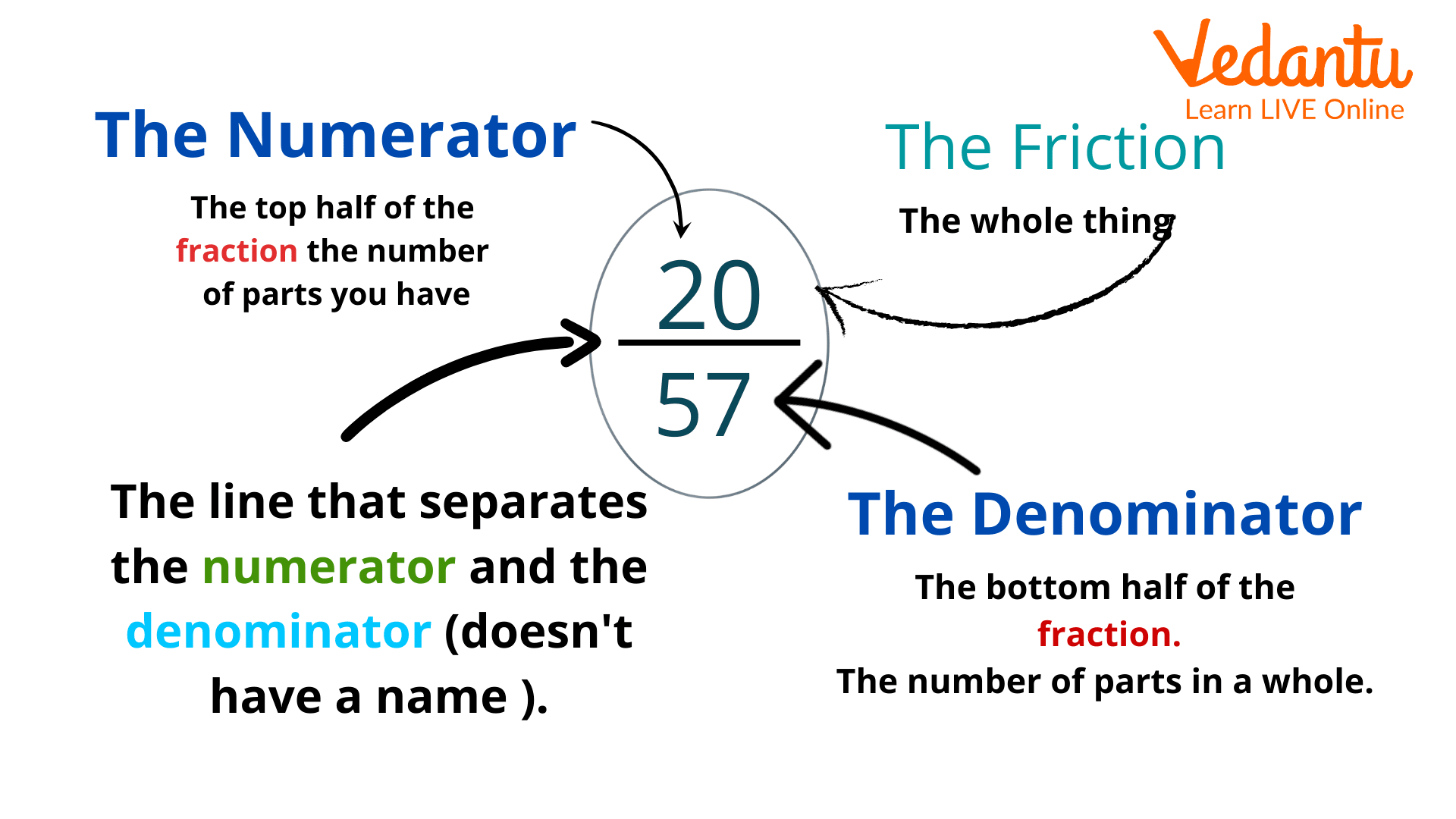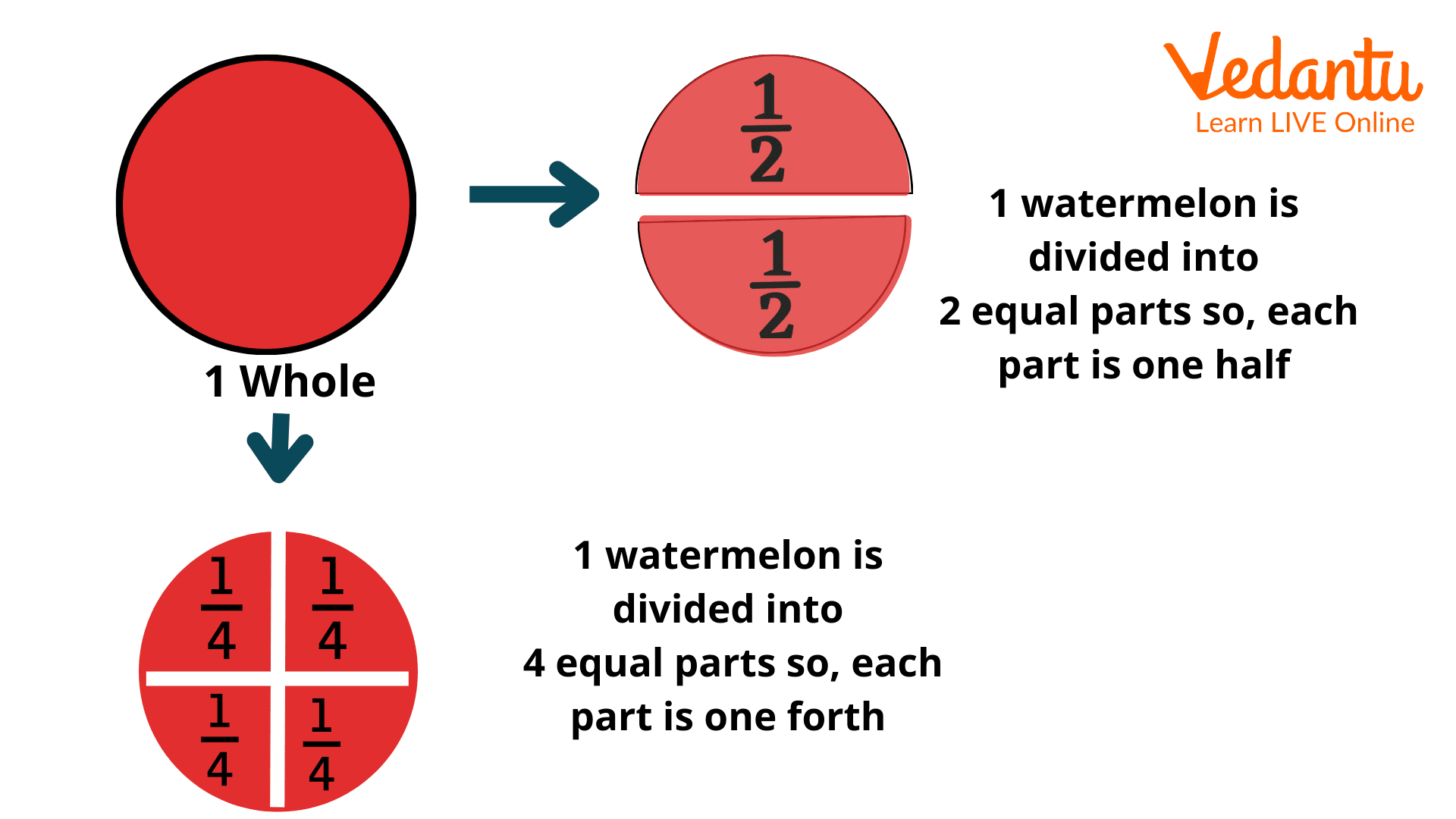Courses
Courses for Kids
Free study material
Free LIVE classes
More

# Learning About Terms Like Halves, Third, and FourthLIVE
Join Vedantu’s FREE Mastercalss

## Introduction to Fractions

Fractions are commonly used terms in Mathematics. As kids progress towards higher grades, they are introduced to several terms; examples of such terms include fraction, halves, thirds, and fourth. Now it is normal to wonder how much fourth is or what half mean. But to understand these terms, we must first understand the concept of fractions. Fraction is defined as the part of the complete set. The fraction consists of two parts: the numerator and the denominator. The terms like halves, thirds, and fourths are commonly used fractions. The concept of fractions can be better understood by the image given below.The fraction parts – the numerator and denominator

The article is focused on the discussion of particular fractions like one-fourth and halves. The article also answers questions like how much is fourth.

## How Much is a Half?

We have already understood that half, thirds, and fourths are the commonly used fractions. Let us now understand what is a half or precisely how much is half. The term half Mathematically represents the value of 12. The fraction is also known as a quarter. Now to calculate how much is a fourth, one has to divide the given number by 4. Before discussing an example of one-fourth calculation, let us look into an image to better understand the fraction.How much is a fourth

Suppose a watermelon weighs 8 kilograms, what is the weight of one-fourth of watermelon?

Since the total weight of watermelon is 8 kgs, the fourth weight can be calculated by dividing the total weight by 4.

84=2

The one-fourth of 8 kgs is 2 kgs.

This was the complete discussion on halves, thirds, and fourths. We have learnt that these terms are commonly used fractions. We have also learnt how to calculate the value of one-thirds, halves, and one-fourths. We hope that we helped in creating a better understanding of the concept. Kids can practise several questions to master the concept of fractions.

Last updated date: 26th Sep 2023
Total views: 138k
Views today: 3.38k

## FAQs on Learning About Terms Like Halves, Third, and Fourth

1. What is a quarter?

Quarter is also a term that is used to represent the value of 1/4 . The term is more commonly used to define time in an analogue watch. A quarter with respect to time represents the time interval of 15 mins. A quarter past 6, refers to the  15 mins past 6, which represents  6:15.

2. What is a fifth of a fraction?

The one-fifth of a fraction represents the Mathematical value of 1/5 . It can be calculated by dividing a given number by 5. As an example, the one-fifth of 10 is 2 as  10/2 is equal to 5.